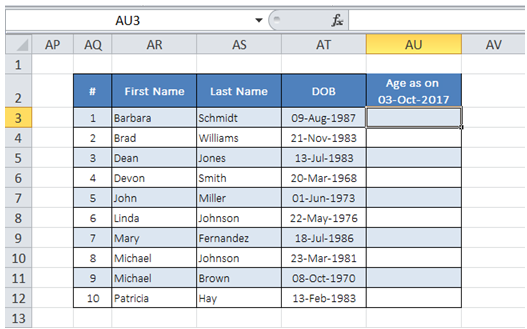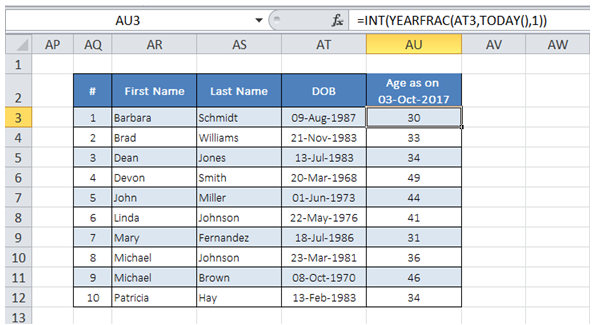## Update current age of customer in table in Excel

In the Table below, I would like to see the current Age updated automatically in col AU.To do it in Excel, here is the answer:

a) Enter the formula =INT(YEARFRAC(AT3,TODAY(),1)) for first client as shown below. Drag the formula to rest of the client column.

TODAY() function used in formula always corresponds to the current date.

YEARFRAC function determines the years since Date of Birth (till date represented by Today()). Third argument "1" defines" normal year calculation (not 360 days).

Since Age corresponds to number of full years, INT function is used to wrap the YEARFRAC function.